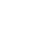kVA to Amperes Conversion Calculator – with Examples

2
3038Last Updated on January 18, 2022 by Krunal Shah (Mod)

With the help of this calculator you can find rated current of device. You can find amperes of both single phase and three phase equipment kVA is known to you.

 .specialclass lblTest { margin-left: 200px; }

How to use the calculator?

• From the drop down, select the phase.
• For this calculator, note down the voltage and kVA of the equipment. You can find details from the equipment’s nameplate.
• Enter the data collected in respective fields.
• The calculator would show automatically the calculated value.

Formulas for kVA to Amperes calculations

Single Phase

For single phase, amperes (I) is equal to kilo volt-amperes (kVA) multiply by 1000 whole divided by Voltage (V). Multiplying by thousand would convert kilo volt-amperes into volt-amperes.Example: Let us say you have 50 kVA transformer with LV side rated voltage is 230 VAC  input current of transformer is 43.478 amps.

Three Phase

For three phase, amperes (I) is equal to kilo volt-amperes (kVA) multiply by 1000 whole divided by Voltage (V) multiply by square root of 3.Example: Let us say we have 5000 kVA transformer with LV side rated voltage is 6.6 kV then the rated current of transformer at LV side is 757.576 amps.

1.win row
•Krunal Shah